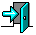# CS3013 Homework 1

Due date: Monday, September 8th

You must turn in your homework in written (or typed) form by the beginning of class on the date it is due.

The questions are based on material presented in class and reading material from the text.

1. Briefly describe the two primary functions of an OS. Give one example for each function.

1. Monolithic kernel
2. Micro kernel
3. Virtual machine

3. Briefly describe how a system call is different from a user level function call. Then, briefly describe the steps involved in a system call in terms of how they are invoked.

4. How does a shell offer the capability to execute program in foreground and background modes. Explain in detail what happens to a process running in the background mode in relation to the shell itself.

5. Consider the following program:

```   main() {
int i;
if (fork()) {
wait(0);
} else {
for(i=0; i<10; i++) {
execl("/bin/ls", "/bin/ls", NULL);
}
}
}
```
1. Assume that the above program is compiled and run. Describe in detail the states and transitions that the process(es) goes through. If there is more than one process, you can assume any order of execution that you wish. Here is a start:
• The process is created and enters the system.
• The process is in the ready state.
• ...
2. What are all the possible outcomes of the run?

6. Given the following set of processes with their CPU burst times:

```   Process       Burst Time
A             5
B             7
C             4
```

Assume processes arrive in the order A, B, C at times 0, 1, and 2 respectively. For each scheduling algorithm below, draw a Gantt chart and compute the average waiting time for:

1. FCFS
2. SJFReturn to the CS3013 Home Page

Send all questions to the TA mailing list.